• 中序遍历二叉排序树 输入一整数序列，建立二叉排序树，然后中序遍历。 输入说明 输入第一行为整数的个数n，第二行是具体的n个整数。 输出说明 建立二叉排序树，然后输出中序遍历的结果。 输入样例 5 1 6 5 9 8 ...数据结构
• 中序遍历二叉排序树 输入一整数序列，建立二叉排序树，然后中序遍历。 输入第一行为整数的个数n，第二行是具体的n个整数。 建立二叉排序树，然后输出中序遍历的结果。 输入示例： 5 1 6 5 9 8 输出： 1 5 6 8 9 #...
中序遍历二叉排序树
输入一整数序列，建立二叉排序树，然后中序遍历。
输入第一行为整数的个数n，第二行是具体的n个整数。
建立二叉排序树，然后输出中序遍历的结果。
输入示例：
5
1 6 5 9 8
输出：
1 5 6 8 9
#include<stdio.h>
#include<stdlib.h>
typedef	struct	node
{
int	data;
struct	node	*left;
struct	node	*right;
}BTnode;

//二叉树的创建
BTnode	*CreateBTree(int	a[],int	n)
{
BTnode	*root,*p,*c,*pa;						//root为根结点	p为开辟新结点	c寻找结点位置	pa为c的前驱
root=(BTnode	*)malloc(sizeof(BTnode));
root->data=a;
root->left=root->right=NULL;
int i;
for(i=1;i<n;i++)
{
p=(BTnode	*)malloc(sizeof(BTnode));
p->data=a[i];
p->left=p->right=NULL;
c=root;
while(c!=NULL)
{
pa=c;
if(c->data>p->data)
c=c->left;
else
c=c->right;
}
if(pa->data>p->data)
pa->left=p;
else
pa->right=p;
}
return	root;
}

//遍历二叉树	中序遍历

void	Inorder(BTnode	*root)
{
if(root)
{
Inorder(root->left);
printf("%d ",root->data);
Inorder(root->right);
}
}
int	main(void)
{

BTnode	*root;
int n,i;
int array;
scanf("%d",&n);
for(i=0;i<n;i++){
scanf("%d",&array[i]);
}
root=CreateBTree(array,n);
Inorder(root);
return	0;
}



展开全文• 代码实现：二叉排序树（BST）的插入、查找（非递归），递归算法、中序遍历二叉排序树，输出有序树，层序遍历BST树、创建一颗二叉排序树、二叉排序树的删除等等。 概念： 没什么好说的，二叉排序树（BST）就是一个...
主题：
代码实现：二叉排序树（BST）的插入、查找（非递归），递归算法、中序遍历二叉排序树，输出有序树，层序遍历BST树、创建一颗二叉排序树、二叉排序树的删除等等。
概念：
没什么好说的，二叉排序树（BST）就是一个中序遍历有序的树，如图中的红色的区域左边整体小于右边，以77为根，明显绿色区域小于黄色区域，
所以进行插入时，遇到了比它的根节点大的，就放在左边，比它的根节点小的就放在右边，然后递归，如果遇到了合适的空位，也就是tr==NULL这时候就是插入的时候，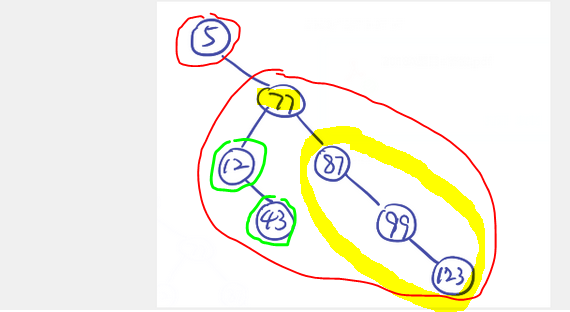难点：
二叉排序树（BST）最难的地方在于其删除操作，

当是需要删除的节点是叶子节点，可以直接删掉，因为它没有左右孩子，不需要考虑删除后，二叉排序树的性质改变。
当需要删除的节点只有一个孩子时，这个孩子可以是左孩子，也可以是右孩子；需要删除当前节点，然后把左孩子（或者右孩子）接到这个被删除节点原本的位置上，也就是被删除节点的父节点的对应孩子变成了被删除节点的孩子，可以理解为【儿子谋权篡位，杀了爸爸，然后继承了爷爷的皇位】 。——————这个从字面上看，需要找到被删除节点的父节点————————而一个巧妙的实现是，交换父节点和儿子节点的值，然后删除儿子节点，并把儿子节点的儿子节点接到原本的父节点的位置。（本文使用的是查找父节点的方式，便于理解）
当需要删除的节点有两个孩子时，删除该节点时，左边都是比该节点小的值，右边都是比该节点大的值，所以需要找到一个合适的值【接替皇位】，这个值可以是正好比该节点小的值（也就是中序遍历的前驱节点）【皇帝的弟弟】，也可以是正好比该节点大的值【皇帝的哥哥】（也就是中序遍历的，后继节点）。这个值作为根节点，显然，这个根节点左边的值都比根节点小，右边的值都比根节点大。————为了实现这个目标，需要一个查找后继节点的函数，并且需要删除原节点，且把接替它的新的根【新皇帝】节点接上去【顶替皇位】。——————一个巧妙的实现方法同上2：交换要删除的值和顶替这个被删除的节点值的值；然后删除这个旧值的节点，这样一来，就转化为了（1或者2或者3）

例如：
删除77，87和77交换位置，而删除77的操作是情况2，需要删除的节点只有一个孩子，这样一来，就可以递归地删除，77的孩子顶替77，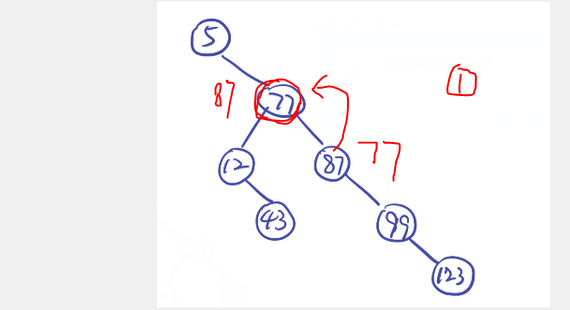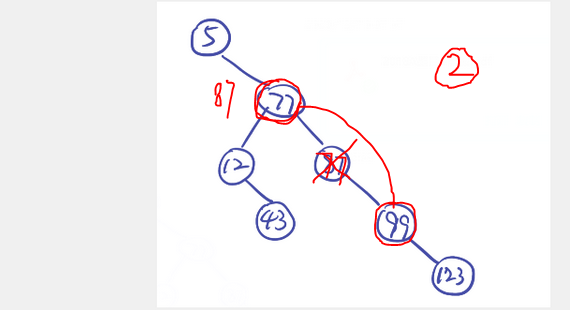得到结果：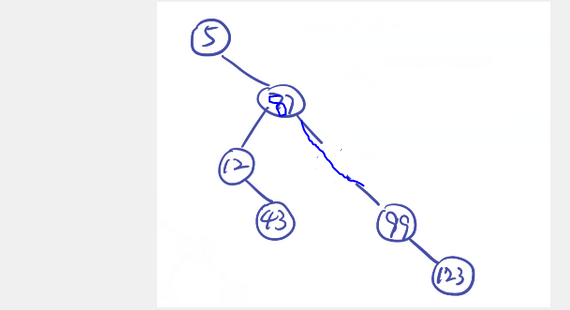输入：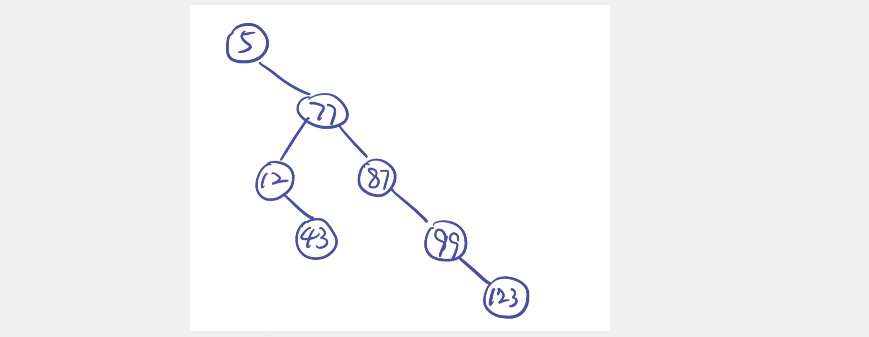5
77
12
87
99
43
123
0

函数调用：
BSTree_use.cpp
#include "BSTree.h"
int main()
{
int key = 5;
BSTree tr = CreateBSTree();
std::cout << "请输入需要查找的值：";
std::cin >> key;

std::cout << "二叉排序树查找" << key << "（非递归）" << BST_Search(tr, key) << std::endl;
std::cout << "二叉排序树查找" << key << "（递归）" << FindNodeK(tr, key) << std::endl;

DeleteBSTreeNode(tr, 77);
DeleteBSTreeNode(tr, 5);
DeleteBSTreeNode(tr, 12);
DeleteBSTreeNode(tr, 87);
DeleteBSTreeNode(tr, 99);
DeleteBSTreeNode(tr, 123);
DeleteBSTreeNode(tr, 43);
}

函数定义：
BSTree.h
#include <iostream>
#include <stack>
#include <queue>
typedef struct BSNode
{
int data;
struct BSNode *lchild, *rchild;
} * BSTree, BSNode;

/***
* 二叉排序树（BST）的插入
* 成功返回1， 失败返回0
*/
int BSTreeInsert(BSTree &tr, int k)
{
if (!tr)
{
tr = (BSNode *)malloc(sizeof(BSNode));
tr->data = k;
tr->lchild = tr->rchild = NULL;
return 1;
}
else if (k == tr->data)
{ //已经有了这个节点了
return 0;
}
else if (k < tr->data)
{
return BSTreeInsert(tr->lchild, k);
}
else
{
return BSTreeInsert(tr->rchild, k);
}
}

/***
* 二叉排序树的查找（非递归）
*/
BSNode *BST_Search(BSTree tr, int k)
{
while (tr && k != tr->data)
{
if (k < tr->data)
tr = tr->lchild;
else
{
tr = tr->rchild;
}
}
return tr;
}

/***
* 二叉排序树（BST）的查找,递归算法
*/
BSNode *FindNodeK(BSTree tr, int k)
{
if (!tr)
return NULL;
FindNodeK(tr->lchild, k);
if (tr->data == k)
return tr;
FindNodeK(tr->rchild, k);
}

/**
* 中序遍历二叉排序树，输出有序树
*/
void VisitBSTree(BSTree tr)
{
if (tr)
{
VisitBSTree(tr->lchild);
std::cout << tr->data << " ";
VisitBSTree(tr->rchild);
}
}
/**
* 层序遍历BST树
*/
void VisitLevelBSTree(BSTree tr)
{
std::queue<BSNode *> q;
BSNode *p = tr, *r = tr;
q.push(p);
while (!q.empty())
{
p = q.front();
q.pop();
if (p)
{
std::cout << p->data << " ";
q.push(p->lchild);
q.push(p->rchild);
if (p == r)
{
std::cout << std::endl;
r = q.back();
}
}
}
}

/**
* 创建一颗二叉树
*/
BSTree CreateBSTree()
{
int input = 5;
BSTree tr = NULL;

std::cout << "请输入树的节点值,输入0结束: ";
std::cin >> input;
while (input)
{
std::cout << BSTreeInsert(tr, input) << " "
<< "目前层序遍历BST树序列：\n";
VisitLevelBSTree(tr);
std::cout << std::endl;
std::cout << "请输入树的节点值,输入0结束: ";
std::cin >> input;
}
return tr;
}

/***
* 二叉排序树的删除
* 1.如果是叶子节点x，直接删除
* 2.如果x只有左儿子，或者只有右儿子，让子树成为被删除节点x的字数，替代x的位置
* 3.节点有左、右两颗子树，令x的后继节点（或者前驱节点）替代x，
*    然后从二叉树删除这个后记节点（或者前驱节点）
* 所以需要额外写一个查找x的后继节点（或者前驱节点）的方法
*/
/***
* 查找节点x的父亲节点的函数
*/
BSNode *FindNodeFather(BSTree tr, int x)
{
std::stack<BSNode *> s;
BSNode *p = tr;
while (p || !s.empty())
{
if (p)
{
s.push(p);
p = p->lchild;
}
else
{
p = s.top();
s.pop();
if ((p->lchild && p->lchild->data == x) || (p->rchild && p->rchild->data == x))
return p;
p = p->rchild;
}
}
return NULL;
}

/**
* 查找BST二叉树的直接后继
*/
BSNode *FindDirectChild(BSTree tr, int x)
{
int next = 0;
std::stack<BSNode *> s;
BSNode *p = tr;
while (p || !s.empty())
{
if (next && p)
return p;
if (p)
{
s.push(p);
p = p->lchild;
}
else
{
p = s.top();
s.pop();

if (p->data == x)
next = 1;
p = p->rchild;
}
}
return NULL;
}

bool DeleteBSTreeNode(BSTree &tr, int x)
{
if (!tr)
return false; //空树，还删除p =。=

BSNode *p = tr;
std::stack<BSNode *> s;
while (!s.empty() || p)
{
if (p)
{
s.push(p);
p = p->lchild;
}
else
{
p = s.top();
if (p->data == x) //找到了这个节点
{
if (!p->lchild && !p->rchild) //是叶子节点，直接删除
{
std::cout << "删除叶子节点 " << x << std::endl;
BSNode *tmp = FindNodeFather(tr, x);
if (!tmp)
{
//返回了空值，说明没有父节点，这个叶子节点是根节点
tr = NULL;
return true;
}
else
{
if (tmp->lchild && tmp->lchild == p)
tmp->lchild = NULL;
else if (tmp->rchild && tmp->rchild == p)
tmp->rchild = NULL;
else
{
std::cout << "-----代码写错了，删除叶子出现了不可能出现的情况-----";
}
}
free(p);
VisitBSTree(tr);
std::cout << std::endl;
return true;
}
else if (p->lchild && p->rchild)
{
std::cout << "删除双孩子节点 " << x << std::endl;
BSNode *t = FindDirectChild(tr, x);
if (t)
{
std::cout << "找到" << x << "后继节点：" << t->data << std::endl;
int deleteNum = 1000000;
p->data = t->data;
t->data = deleteNum;
DeleteBSTreeNode(tr, deleteNum);
}
else
{
std::cout << "-----代码写错了，删除双孩子出现了不可能出现的情况-----";
}
return false;
}
else if (!p->lchild || !p->rchild)
{

std::cout << "删除单孩子节点 " << x << std::endl;
BSNode *child;
if (p->lchild)
child = p->lchild;
else
child = p->rchild;
BSNode *tmp = FindNodeFather(tr, x);
if (!tmp)
{
//返回了空值，说明没有父节点，这个单孩子节点是根节点
tr = child;
std::cout << "删除根孩子节点，更新根节点为： " << tr->data << std::endl;
}
else
{
if (tmp->lchild && tmp->lchild == p)
tmp->lchild = child;
else if (tmp->rchild && tmp->rchild == p)
tmp->rchild = child;
else
{
std::cout << "-----代码写错了，删除单孩子出现了不可能出现的情况-----";
}
}
free(p);
VisitBSTree(tr);
std::cout << std::endl;
return true;
}
else
{
std::cout << "-----代码写错了，删除出现了不可能出现的情况-----";
}
return false;
}
s.pop();
p = p->rchild;
}
}
return false; //没有这个节点，直接返回了
}


碎碎念
实现大概花了4个小时，比较慢，有些地方实在不能立刻理解，好在最后全部实现了 = = 。
写得不错的参考：
动态规划 - 最优二叉搜索树


展开全文数据结构 算法 链表 c++
• 输入一整数序列，建立二叉排序树，然后中序遍历。 输入说明 输入第一行为整数的个数n，第二行是具体的n个整数。 输出说明 建立二叉排序树，然后输出中序遍历的结果。 输入样例 5 1 6 5 9 8 输出样例 1 5 6 8 9 #...
问题描述
输入一整数序列，建立二叉排序树，然后中序遍历。
输入说明
输入第一行为整数的个数n，第二行是具体的n个整数。
输出说明
建立二叉排序树，然后输出中序遍历的结果。
输入样例
5
1 6 5 9 8
输出样例
1 5 6 8 9
#include<stdio.h>
#include<stdlib.h>
typedef struct node
{
int key;
struct node *lchild,*rchild;
}bst;
bst *root;
bst *insert(bst *t,bst *s)
{
bst *f,*p;
p=t;
while(p!=NULL)
{
f=p;
if(s->key==p->key) return t;
else if(s->key<p->key) p=p->lchild;
else p=p->rchild;
}
if(t==NULL) return s;
if(s->key<f->key) f->lchild=s;
else f->rchild=s;
return t;
}
bst *tree(int k[],int n)
{
int i;
bst *t,*s;
t=NULL;
for(i=0;i<n;i++)
{
s=(bst*)malloc(sizeof(bst));
s->lchild=NULL;s->rchild=NULL;
s->key=k[i];
t=insert(t,s);
}
return t;
}
void inorder(bst *p)
{
bst *stack;
bst *s;
s=p;
int top=-1;
while(top!=-1||s!=NULL)
{
while(s!=NULL)
{
top++;
stack[top]=s;
s=s->lchild;
}
s=stack[top];
top--;
printf("%d ",s->key);
s=s->rchild;
}
}
int main()
{
root=(bst*)malloc(sizeof(bst));
int n,i;
scanf("%d",&n);
int k[n];
for(i=0;i<n;i++) scanf("%d",&k[i]);
root=tree(k,n);
inorder(root);
return 0;
}



展开全文• 通过中序遍历二叉搜索树得到的关键码序列是一个递增序列。 这是二叉搜索的一个重要性质，巧妙利用这一性质可以解决一系列二叉搜索问题。 本系列以以下非递归中序遍历代码为核心，解决一系列相关问题。 p = ...
中序遍历解决二叉搜索树问题

Python3

树

深度优先搜索

通过中序遍历二叉搜索树得到的关键码序列是一个递增序列。
这是二叉搜索树的一个重要性质，巧妙利用这一性质可以解决一系列二叉搜索树问题。
本系列以以下非递归中序遍历代码为核心，解决一系列相关问题。

p = root
st = []  # 用列表模拟实现栈的功能
while p is not None or st:
while p is not None:
st.append(p)
p = p.left
p = st.pop()
proc(p.val)
p = p.right


一 二叉搜索树迭代器
（一）算法思路
中序遍历二叉树
（二）算法实现

class BSTIterator:

def __init__(self, root: TreeNode):
self.root = root
self.st = []
self.current = self.root

def next(self) -> int:
"""
@return the next smallest number
"""
while self.current is not None or self.st:
while self.current is not None:
self.st.append(self.current)
self.current = self.current.left
self.current = self.st.pop()
node = self.current
self.current = self.current.right
return node.val

def hasNext(self) -> bool:
"""
@return whether we have a next smallest number
"""
return self.current or self.st


二 二叉搜索树的最小绝对差
（一）算法思路
中序遍历二叉搜索树，第一个结点外的每个节点与其前一节点的差值，如果该值小于最小绝对差，就用它更新最小绝对差（初始可设为无穷）。
（二）算法实现

class Solution:
def getMinimumDifference(self, root: TreeNode) -> int:
st = []
p = root
pre = -float('inf')
min_val = float('inf')
while p is not None or st:
while p is not None:
st.append(p)
p = p.left
p = st.pop()
cur = p.val
if cur - pre < min_val:
min_val = cur - pre
pre = cur
p = p.right
return min_val


（三）复杂度分析
时间复杂度：O(N)，N为树中节点个数。
空间复杂度：O(log(N))。

三 二叉搜索树中第k小的元素
（一）算法思路
二叉搜索树的中序遍历序列为递增序列，因此可中序遍历二叉搜索树，返回第K个元素。
（二）算法实现

# Definition for a binary tree node.
# class TreeNode:
#     def __init__(self, x):
#         self.val = x
#         self.left = None
#         self.right = None

class Solution:
def kthSmallest(self, root: TreeNode, k: int) -> int:
st = []
p = root
s = 0
while p is not None or st:
while p is not None:
st.append(p)
p = p.left
p = st.pop()
s += 1
if s == k:
return p.val
p = p.right


（三） 复杂度分析
时间复杂度：O(N)，N为树中节点个数。
空间复杂度：O(log(N))。

四 二叉搜索树中的众数
（一） 算法思想
二叉搜索树的中序遍历序列单调不减（或曰非严格单调递增），因此可考虑中序遍历二叉搜索树。
用max_times记录已访问节点的最大重复元素个数，time表示当前访问节点的元素值的出现次数,用res=[]记录结果。
若time == max_times，则将当前节点值添加到结果集。
若time > max_times，则以当前节点值构造新的列表作为结果，并用time更新max_times。
中序遍历结束后，返回结果res。
（二） 算法实现

class Solution:
def findMode(self, root: TreeNode) -> List[int]:
if root is None:
return []
p = root
st = []
res = []
max_times = 1
time = 1
pre = float("inf")
while p is not None or st:
while p is not None:
st.append(p)
p = p.left
p = st.pop()

cur = p.val
if cur == pre:
time += 1
else:
time = 1
pre = cur
if time == max_times:
res.append(cur)
if time > max_times:
res = [cur]
max_times = time

p = p.right

return res


（三） 复杂度分析
时间复杂度：O(N)，N为树中节点个数。
空间复杂度：最坏情况下为O(N)， 例如树畸形（树的高度为线性）或每个元素出现一次的情形。

五 二叉搜索树的范围和
（一）算法思路
中序遍历二叉搜索树
当节点的值等于L时开始累加，当节点的值等于R时停止累加并返回累加的结果。
（二）算法实现

class Solution:
def rangeSumBST(self, root: TreeNode, L: int, R: int) -> int:
st = []
p = root
s = 0
while p is not None or st:
while p is not None:
st.append(p)
p = p.left
p = st.pop()
if p.val == L:
s = L
p = p.right
break
p = p.right

while p is not None or st:
while p is not None:
st.append(p)
p = p.left
p = st.pop()
s += p.val
if p.val == R:
return s
p = p.right


（三）复杂度分析
时间复杂度：O(N), N为树中节点数。
空间复杂度：O(log(N))。

六 两数之和IV-输入BST
（一）算法思路
中序遍历+双指针
（二）算法实现

class Solution(object):
def findTarget(self, root, k):
"""
:type root: TreeNode
:type k: int
:rtype: bool
"""
nums = []
st = []
p = root
while p is not None or st:
while p is not None:
st.append(p)
p = p.left
p = st.pop()
nums.append(p.val)
p = p.right

n = len(nums)
i, j = 0, n-1
while i < j:
if nums[i] + nums[j] == k:
return True
elif nums[i] + nums[j] > k:
j -= 1
else:
i += 1
return False


（三）复杂度分析
时间复杂度：O(N)
空间复杂度：O(N)

七 验证二叉搜索树
（一）算法思路
一棵二叉树是二叉搜索树的充要条件是它的中序遍历序列单调递增，因此可以通过判断一个树的中序遍历序列是否单调递增来验证该树是否为二叉搜索树。
（二）算法实现

# Definition for a binary tree node.
# class TreeNode(object):
#     def __init__(self, x):
#         self.val = x
#         self.left = None
#         self.right = None

class Solution(object):
def isValidBST(self, root):
"""
:type root: TreeNode
:rtype: bool
"""
pre = -float('inf')
p = root
st = []
while p is not None or st:
while p is not None:
st.append(p)
p = p.left
p = st.pop()
if p.val > pre:
pre = p.val
else:
return False
p = p.right
return True

展开全文• !... 我看书上说“二叉排序树中序遍历结果是递增序列”，然后我随便写了几个数，生成二叉排序树，对其进行中序遍历，结果如上图，它不是递增序列啊？？？是我知识上有什么漏洞吗？求教啊~~
• 实现折半查找与遍历二叉排序树 一、实验目的 掌握几种典型的查找方法（折半查找、二叉排序树的查找、哈希查找）。 对各种查找算法的特点、使用范围和效率有进一步的了解。 了解图的典型应用的算法程序。 二、实验...算法 数据结构 c语言
• 二叉树是一种非常重要的数据结构，它同时具有数组和链表各自的特点：它可以像数组一样快速查找，也可以像...对于排序二叉树，若按中序遍历就可以得到由小到大的有序序列。 我们先介绍一些关于二叉树的概念名词。 ...
• 根据输入数据建造二叉排序树，并中序遍历，从而输出结果
• * 二叉排序树BST的插入和中序遍历 * 排序树的中序遍历输出序列是递增的 **/ #include<iostream> using namespace std; #define DataType int #define test_length 12 DataType test_data[test_length]={5,...c++ 数据结构
• 二叉排序树的定义 二叉排序树，也称二叉查找树。二叉排序树或者是一棵空树，或者是一颗具有以下特性的非空二叉树： ...右子树结点值，对二叉树中序遍历得到一个递增的有序序列二叉排序树的创建...数据结构
• 可能有多组测试数据，对于每组数据，将题目给数据建立一个二叉排序树，并对二叉排序树进行前序、中序和后序遍历。每种遍历结果输出一行。每行最后一个数据之后有一个空格。 样例输入 1 2 2 8 15 4 21 10 5 39 ...
• 二叉排序树的实现 二叉链表作存储结构 ...2) 对二叉排序树T作中序遍历，输出结果； 3) 输入元素x,查找二叉排序树T,若存在含x的结点,则删除该结点, 并作中序遍历(执行操作2)；否则输出信息“无x”
• 结构练习——排序二叉树的中序遍历 Problem Description 在结构中，有一种特殊的二叉树叫做排序二叉树，直观的理解就是——(1).每个节点中包含有一个关键值 (2).任意一个节点的左子树（如果存在的话）的关键值...
• 排序二叉树的中序遍历 Problem Description 在结构中，有一种特殊的二叉树叫做排序二叉树，直观的理解就是——(1).每个节点中包含有一个关键值 (2).任意一个节点的左子树（如果存在的话）的关键值小于该节点...
• tree = input ( ) . split ( ' ' ) cen = tree [ 0 ] #print(cen) after = tree [ 1 ] pre = [ ] def ...#Python join() 方法用于将序列中的元素以指定的字符连接生成一个新的字符串。python
• 二叉排序树创建、中序遍历（由小到大）、交换左右子树输出（由大到小），完整C++实现代码，在Clion中编译通过。 #include "stdio.h" #include "stdlib.h" #include "malloc.h" //...C++ 交换左右子树
• 题目：建立二叉排序树，输出其先序遍历，中序遍历，后序遍历 样例输出： 样例输出： 实现代码： #include struct Node{ Node *lchild; Node *rchild; int x; }Tree; int loc; Node *Create...
• #include <iostream> #include <cstdio> #include <queue> using namespace std;...struct TreeNode{ ...TreeNode* Insert(TreeNode* root, int x){//二叉排序树建树 if(root == NULL){
• 判断是否是排序二叉树只需要在中序遍历的过程中判断这次的遍历结果是否比上次的大即可。 查看此序列是否是二叉搜索的后序遍历 很显然，二叉搜索是按照中序排列从小到大，那它的后序遍历就是小大小的顺序。 1....数据结构 leetcode java 算法
• 概述： ...点，这个时候我们就引入了二叉排序树。 树结构查找是将查找表按照某种规律建成树结构。因为建构的树结构是按某种规律建立的，因此查找 过程也遵循这种规律，可以获得较高的查找效率。 1、...
• 二叉树的非递归中序遍历 中序序列为左根右，因此用栈模拟这一过程，就是从当前子树根结点出发，一直往左走，把沿途结点全部入栈，直到最左边，对于最左边的结点来说，他没有左孩子，根据“左根右”的顺序，这时需要...
• 二叉搜索中序遍历也可以实现排序 package org.exam.ch8; /** * Created by xin on 15.10.14. */ class Node{ public int iData; public double dData; public Node leftChild; public Node rightChild; ...
• package sortTest; // 节点 class treeNode{ int data; treeNode left = null; treeNode right = null; public treeNode(int data) { this.data = data; } } public class sortT...
• 完全二叉树的层序中（从1开始），根为root，左子树若存在则为2*root，右子树为2*root+1。...二叉排序树的中序序列是非递减的，sort以后即可得到中序序列。结合中序遍历，第一次执行到visit（root）是最小的结...
• Problem：给出n个int数，对其构造一棵二叉排序树，并打印其先序遍历、中序遍历、后序遍历的一维数组。 （华中科技大学保研机试真题） #include &lt;stdio.h&gt; #include &lt;string.h&gt; ...数据结构
• 编译器：Xcode 编程语言：Cdata1.txt文本数据为上图，在我的电脑里它的存储位置是：/Users/wsw/Desktop/数据结构/data1.txt#include ...#define N 100typedef struct node //二叉排序树结点定义 { int key;数据结构
• #include<iostream> using namespace std; typedef struct node { int data; node* lChild, * rChild; }Tree,*Bitree; void CreateTreeByOrder(Bitree& t, int n) { if (t) { ...data &...
• 给定一个二叉树，判断它是否是合法的二叉查找(BST) 一棵BST定义为： 节点的左子树中的值要严格小于该节点的值。节点的右子中的值要严格大于该节点的值。左右子树也必须是二叉查找。一个节点的也是二叉查找...算法...